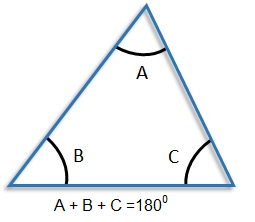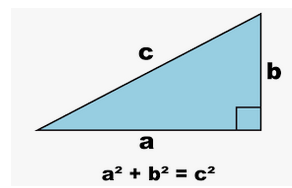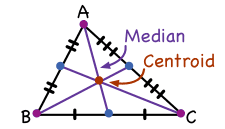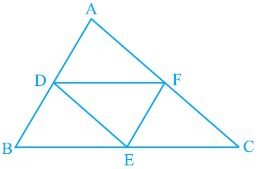[Updated] Goldman Sachs Aptitude Test Questions and Answers
Practice List of TCS Digital Coding Questions !!!
Take 50+ FREE!! Online Data Interpretation Mock test to crack any Exams.

# Quantitative Aptitude :: Area

Home > Quantitative Aptitude > Area > Important Formulas

## Area Important Formulas

1. Results on Triangles:A triangle is a polygon with three sides and three corners. It is one of the basic shapes in geometry. The corner is a triangle with A, B, and C. In Euclidean geometry, any three points, when non-collinear, determine a unique triangle as well as a unique plane.

$$\mathbb{i.}$$ Sum of the angles of a triangle is $$180^{0}$$.

$$\mathbb{ii.}$$The sum of any two sides of a triangle is greater than the third side.(i.e A+B > C or B+C > A etc.)In mathematics, the Pythagoras theorem, also known as the Pythagoras theorem, is a fundamental relation in Euclidean geometry between three sides of a right triangle. This states that the area of the square whose side is the hypotenuse is equal to the sum of the squares on the other two sides.

$$\mathbb{iii.}$$In a right-angled triangle, $$Hypotenuse^{2}$$ = $$Base^{2}$$ + $$Height^{2}$$.$$\mathbb{iv.}$$ The line joining the mid-point of a side of a triangle to the positive vertex is called the median.

$$\mathbb{v.}$$ The point where the three medians of a triangle meet, is called centroid. The centroid divided each of the medians in the ratio 2 : 1.

$$\mathbb{vi.}$$ In an isosceles triangle, the altitude from the vertex bisects the base.

$$\mathbb{vii.}$$ The median of a triangle divides it into two triangles of the same area.$$\mathbb{viii.}$$ The area of the triangle formed by joining the mid-points of the sides of a given triangle is one-fourth of the area of the given triangle.A quadrilateral (quad means four, lateral means side) is a polygon with four sides. There are seven quadrants, some that are definitely familiar to you, and some that are not so familiar. Look at the following definition and the quadrilateral family tree in the following figure.

Kite: A quadrilateral in which two disjoint pairs of consecutive sides are congruent ("disjoint pairs" means that one side can’t be used in both pairs).

Parallelogram: A quadrilateral that has two pairs of parallel sides.

Rhombus: A quadrilateral with four congruent sides; a rhombus is both a kite and a parallelogram.

Rectangle: A quadrilateral with four right angles; a rectangle is a type of parallelogram.

Square: A quadrilateral with four congruent sides and four right angles; a square is both a rhombus and a rectangle.

Trapezoid: A quadrilateral with exactly one pair of parallel sides (the parallel sides are called bases).

Isosceles trapezoid: A trapezoid in which the nonparallel sides (the legs) are congruent.

Important Points:

$$\mathbb{i.}$$ The diagonals of a parallelogram bisect each other.

$$\mathbb{ii.}$$ Each diagonal of a parallelogram divides it into triangles of the same area.

$$\mathbb{iii.}$$ The diagonals of a rectangle are equal and bisect each other.

$$\mathbb{iv.}$$ The diagonals of a square are equal and bisect each other at right angles.

$$\mathbb{v.}$$ The diagonals of a rhombus are unequal and bisect each other at right angles.

$$\mathbb{vi.}$$A parallelogram and a rectangle on the same base and between the same parallels are equal in area.

$$\mathbb{vii.}$$ Of all the parallelogram of given sides, the parallelogram which is a rectangle has the greatest area.

3. IMPORTANT FORMULAE:$$\mathbb{1.}$$ Area of a rectangle = $$\left(Length \times Breadth \right)$$.
Length = $$\left( \frac{Area}{Breadth}\right)$$ and Breadth = $$\left(\frac{Area}{Length}\right)$$.

$$\mathbb{2.}$$ Perimeter of a rectangle = $$2 \left( Length + Breadth \right)$$.Area of a square = $$\left(side\right)^{2}$$ = $$\frac{1}{2}(diagonal)^{2}$$.Area of 4 walls of a room = $$2 \left( Length + Breadth \right) \times Height$$.$$\mathbb{1.}$$ Area of a triangle = $$\frac{1}{2} \times Base \times Height$$.

$$\mathbb{2.}$$ Area of a triangle = $$\sqrt{s(s-a)(s-b)(s-c)}$$.
where a, b, c are the sides of the triangle and s = $$\frac{1}{2}\left(a + b + c \right)$$.

$$\mathbb{3.}$$ Area of an equilateral triangle = $$\frac{\sqrt{3}}{4} \times (sides)^{2}$$.

$$\mathbb{4.}$$ Radius of incircle of an equilateral triangle of side a = $$\frac{a}{2\sqrt{3}}$$

$$\mathbb{5.}$$ Radius of circumcircle of an equilateral triangle of side a = $$\frac{a}{\sqrt{3}}$$

$$\mathbb{6.}$$ Radius of incircle of a triangle of area $$\Delta$$ and semi-perimeter r = $$\frac{\Delta}{s}$$$$\mathbb{1.}$$ Area of parallelogram = $$\left( Base \times Height \right)$$.

$$\mathbb{2.}$$ Area of a rhombus = $$\frac{1}{2} \times \left( Product \ of \ diagonals \right)$$.

$$\mathbb{3.}$$ Area of a trapezium = $$\frac{1}{2} \times \left( sum \ of \ parallel \ sides \right) \times \left( distance \ between \ them \right)$$.$$\mathbb{1.}$$ Circumference of a semi-circle = $$\pi R$$, where R is the radius and $$\pi$$ = 3.14159265359 = ~3.15.

$$\mathbb{2.}$$ Area of semi-circle = $$\frac{\pi R^{2}}{2}$$.